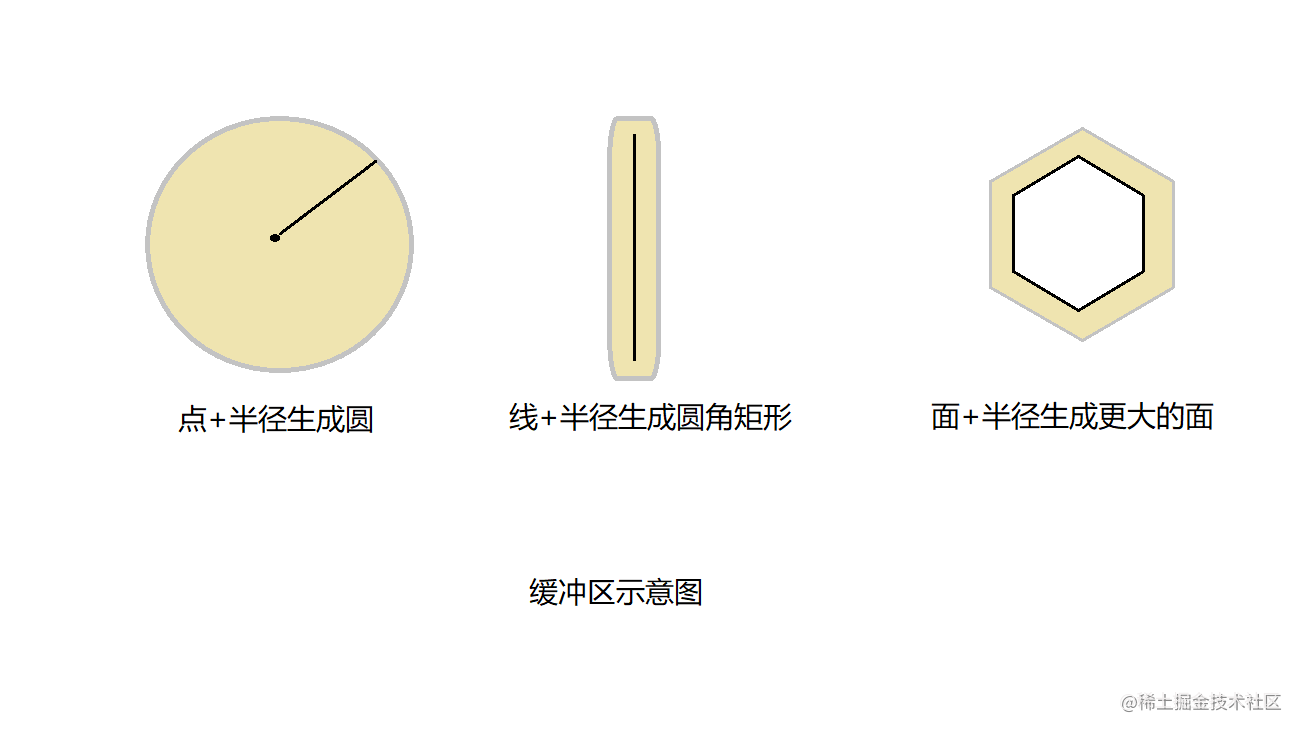# 数据类型

## 1.什么是MySQL空间数据

• MySQL提供了数据类型geometry用来存储坐标信息，geometry类型支持以下三种数据存储

POINT(点)POINT(113.3 40.08)用于存储点位信息，包含经纬度信息
LINESTRING(线)LineString(84.070 33.801,99.52 30.292)用来存储路线信息
POLYGON(面)POLYGON((84.070 33.801, 84.100 33.801,84.070 33.801))用来存储面数据

## 2.什么是geojson

GeoJSON是一种对各种地理数据结构进行编码的格式。GeoJSON对象可以表示几何、特征或者特征集合。GeoJSON支持下面几何类型：点、线、面、多点、多线、多面和几何集合。GeoJSON里的特征包含一个几何对象和其他属性，特征集合表示一系列特征。一个完整的GeoJSON数据结构总是一个（JSON术语里的）对象。在GeoJSON里，对象由名/值对--也称作成员的集合组成。对每个成员来说，名字总是字符串。成员的值要么是字符串、数字、对象、数组，要么是下面文本常量中的一个："true","false"和"null"。数组是由值是上面所说的元素组成

{ "type": "Point", "coordinates": [经度, 纬度] }

{"type":"Polygon","coordinates":[[[经度,纬度],[经度,纬度],[经度,纬度]]]}

## 3.格式化空间数据类型（geometry相互转换geojson）

• 数据库存储的空间数据通过可视化工具展示的明文结构为上面示例中所见，结构并不易于客户端解析，所以MySQL提供了几个空间函数用来解析及格式化空间数据，geojson是gis空间数据展示的标准格式，前端地图框架及后端空间分析相关框架都会支持geojson格式

geojson -> geometryST_GeomFromGeoJSON
geometry -> geojsonST_ASGEOJSON
geometry字符串 -> geometryST_GEOMFROMTEXT

### 示例

#### 准备示例数据

id(bigint)point_name(varchar)point_geom(geometry)
1绿藤气象监测点POINT(116.410671499 40.1849142015)
2长藤资本监测点POINT(113.3 40.08)

#### 函数应用示例

1.查询绿藤气象监测点信息将geometry处理成geojson格式

``````select id,point_name,ST_ASGEOJSON(point_geom) as geojson from meteorological_point where id = 1

idpoint_namegeojson
1绿藤气象监测点{"type": "Point", "coordinates": [116.410671499, 40.1849142015]}

2.新增一个点位信息，客户端提交的点位geometry字符串需要使用ST_GEOMFROMTEXT函数处理才能插入，否则会报错

``````{
"point_name":"新帅集团监测点",
"geotext":"POINT(117.420671499 40.194914201)"}
}

``````insert into meteorological_point(point_name, point_geom) values("新帅集团监测点", "POINT(117.420671499 40.194914201)")

``````insert into meteorological_point(point_name, point_geom) values("新帅集团监测点", ST_GEOMFROMTEXT("POINT(117.420671499 40.194914201)"))

3.新增点位，客户端提交点位格式为geojson格式，需要使用ST_GeomFromGeoJSON函数处理后进行插入

``````{
"point_name":"民爆公司监测点",
"geojson":"{"type": "Point", "coordinates": [117.410671499, 40.1549142015]}"}
}

``````insert into meteorological_point(point_name, point_geom) values("民爆公司监测点", ST_GeomFromGeoJSON("{\"type\": \"Point\", \"coordinates\": [117.410671499, 40.1549142015]}"))

#### 空间数据格式化小结

mysql geometry数据存储需要对geometry文本或geojson进行函数处理后才能进行存储，否则会报错，查询时候使用格式化函数转成geojson方便服务端传输和客户端框架解析

# 空间分析

## 根据点位及半径，生成缓冲区``````SELECT ST_ASGEOJSON(ST_BUFFER(ST_GeomFromGeoJSON('\${geojsonStr}'),\${radius}))

### 示例

1.有一个点位的geojson字符串为 "{"type": "Point", "coordinates": [117.410671499, 40.1549142015]}",缓冲半径50米（注意：ST_BUFFER()的参数地理信息及返回值均使用墨卡托坐标系，如非墨卡托坐标系的geojson,需使用工具类进行转换处理）

``````public class MercatorUtils {
/**
* 点位geojson转墨卡托
*
* @param point
* @return
*/
public static JSONObject point2Mercator(JSONObject point) {
JSONArray xy = point.getJSONArray(COORDINATES);
JSONArray mercator = lngLat2Mercator(xy.getDouble(0), xy.getDouble(1));
point.put(COORDINATES, mercator);
return point;
}
/**
* 经纬度转墨卡托
*/
public static JSONArray lngLat2Mercator(double lng, double lat) {
double x = lng * 20037508.342789 / 180;
double y = Math.log(Math.tan((90 + lat) * M_PI / 360)) / (M_PI / 180);
y = y * 20037508.34789 / 180;
JSONArray xy = new JSONArray();
return xy;
}

/**
* 墨卡托坐标系数据转普通坐标系
*/
public static JSONObject mercatorPolygon2Lnglat(JSONObject polygon) {
JSONArray coordinates = polygon.getJSONArray(COORDINATES);
JSONArray xy = coordinates.getJSONArray(0);
JSONArray ms = new JSONArray();
for (int i = 0; i < xy.size(); i++) {
JSONArray p = xy.getJSONArray(i);
JSONArray m = mercator2lngLat(p.getDouble(0), p.getDouble(1));
}
JSONArray newCoordinates = new JSONArray();
polygon.put(COORDINATES, newCoordinates);
return polygon;
}
}

• 客户端提交点位geojson及半径
• 使用墨卡托工具类将点位geojson转换成墨卡托坐标系的geojson
• 调用sql进行缓冲区生成
• 返回值使用墨卡托工具类转换成mercatorPolygon2Lnglat返回给调用方

## 判断点位所在城市

2.判断用户点位所在城市-客户端提交用户的定位信息，判断用户所在城市(使用ST_INTERSECTS()判断两个几何是否相交即可，返回0或1)

``````SELECT ST_INTERSECTS(ST_GeomFromGeoJSON('\${geoJsonStrA}'), ST_GeomFromGeoJSON('\${geoJsonStrB}'))

# 常用的空间函数

ST_INTERSECTS()判断两个几何是否相交
ST_DISTANCE()两个几何的距离
ST_CONTAIONS()几何是否包含
ST_ISVALID()几何是否有效

# 总结

MySQL为空间数据的存储及分析提供了丰富的数据类型及函数，我们学习此类函数能够帮助我们更好的处理地理信息，使用前需要对坐标系、geojson相关知识进行了解，避免踩坑，如果有相关问题也可以在评论区交流，如有误区请指正。